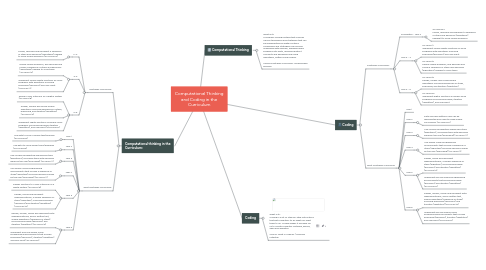# Computational Thinking and Coding in the Curriculum

Get Started. It's FreeComputational Thinking and Coding in the Curriculum## 1. Coding

### 1.1. Australian Curriculum:

1.1.1. Foundation - Year 2

1.1.1.1. ACTDIP004 Follow, describe and represent a sequence of steps and decisions (algorithms) neededÂ to solve simple problems

1.1.2. Year 3 - 4

1.1.2.1. ACTDIP011 Implement simple digital solutions as visual programs with algorithms involving branching (decisions) and user input

1.1.2.2. ACTDIP010 Define simple problems, and describe and follow a sequence of steps and decisions (algorithms) needed to solve them

1.1.3. Year 5 - 6

1.1.3.1. ACTDIP019 Design, modify and follow simple algorithms involving sequences of steps, branching, and iteration (repetition)

1.1.3.2. ACTDIP020 Implement digital solutions as simple visual programs involving branching, iteration (repetition), and user input

### 1.2. West Australian Curriculum

1.2.1. Year 1

1.2.2. Year 2

1.2.2.1. Data can have patterns and can be represented and used to make simple conclusions (ACTDIK002)

1.2.3. Year 3

1.2.3.1. Use visually represented sequenced steps (algorithms), including steps with decisions made by the user (branching) (ACTDIP011)

1.2.4. Year 4

1.2.4.1. Use simple visual programming environments that include a sequence of steps (algorithm) involving decisions made by the user (branching) (ACTDIP011)

1.2.5. Year 5

1.2.5.1. Design, follow and represent diagrammatically, a simple sequence of steps (algorithm), involving branching (decisions) and iteration (repetition) (ACTDIP019)

1.2.5.2. Implement and use simple programming environments that include branching (decisions) and iteration (repetition) (ACTDIP020)

1.2.6. Year 6

1.2.6.1. Design, modify, follow and represent both diagrammatically, and in written text, simple algorithms (sequence of steps) involving branching (decisions) and iteration (repetition) (ACTDIP019)

1.2.6.2. Implement and use simple visual programming environments that include branching (decisions), iteration (repetition) and user input (ACTDIP020)

## 3. Computational thinking in the Curriculum:

### 3.1. Australian Curriculum:

3.1.1. F-2:

3.1.1.1. Follow, describe and represent a sequence of steps and decisions (algorithms) needed to solve simple problems (ACTDIP004)

3.1.2. 3-4:

3.1.2.1. Define simple problems, and describe and follow a sequence of steps and decisions (algorithms) needed to solve them (ACTDIP010)

3.1.2.2. Implement simple digital solutions as visual programs with algorithms involving branching (decisions) and user input (ACTDIP011)

3.1.3. 5-6:

3.1.3.1. Design a user interface for a digital system (ACTDIP018)

3.1.3.2. Design, modify and follow simple algorithms involving sequences of steps, branching, and iteration (repetition) (ACTDIP019)

3.1.3.3. Implement digital solutions as simple visual programs involving branching, iteration (repetition), and user input (ACTDIP020)

### 3.2. West Australian Curriculum:

3.2.1. Year 1

3.2.1.1. Use data to solve a simple task/problem (ACTDIP003)

3.2.2. Year 2

3.2.2.1. Use data to solve similar tasks/problems (ACTDIP003)

3.2.3. Year 3

3.2.3.1. Use visually represented sequenced steps (algorithms), including steps with decisions made by the user (branching) (ACTDIP011)

3.2.4. Year 4

3.2.4.1. Use simple visual programming environments that include a sequence of steps (algorithm) involving decisions made by the user (branching) (ACTDIP011)

3.2.5. Year 5

3.2.5.1. Design solutions to a user interface for a digital system (ACTDIP018)

3.2.5.2. Design, follow and represent diagrammatically, a simple sequence of steps (algorithm), involving branching (decisions) and iteration (repetition) (ACTDIP019)

3.2.6. Year 6

3.2.6.1. Design, modify, follow and represent both diagrammatically, and in written text, simple algorithms (sequence of steps) involving branching (decisions) and iteration (repetition) (ACTDIP019)

3.2.6.2. Implement and use simple visual programming environments that include branching (decisions), iteration (repetition) and user input (ACTDIP020)